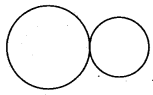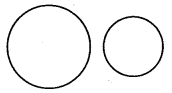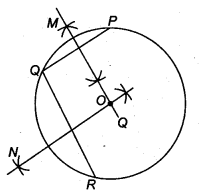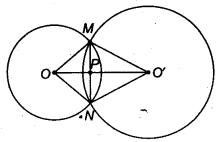NCERT Solutions for Class 9 Maths Chapter 11 Circles Ex 11.3 are part of NCERT Solutions for Class 9 Maths. Here we have given NCERT Solutions for Class 9 Maths Chapter 11 Circles Ex 11.3.

 Board CBSE Textbook NCERT Class Class 9 Subject Maths Chapter Chapter 11 Chapter Name Circles Exercise Ex 11.3 Number of Questions Solved 3 Category NCERT Solutions

## NCERT Solutions for Class 9 Maths Chapter 11 Circles Ex 11.3

Question 1.
Draw different pairs of circles. How many points does each pair have in common? What is the maximum number of common points?
Solution:
Different pairs of circles are
(i) Two points common(ii) One point is common(iii) No point is common(iv) No point is common(v) One point is commonFrom figures, it is obvious that these pairs many have 0 or 1 or 2 points in common.
Hence, a pair of circles cannot intersect each other at more than two points.

Question 2.
Suppose you are given a circle. Give a construction to find its centre.
Solution:
Steps of constructionTaking three points P,Q and R on the circle.
Join PQ and QR,
Draw MQ and NS, respectively the perpendicular bisectors of PQ and RQ, which intersect each other at O.
Hence, O is the centre of the circle.

Question 3.
If two circles intersect at two points, prove that their centres lie on the perpendicular bisector of the common chord.
Solution:
Given: Two circles with centres O and O’ intersect at two points M and N so that MN is the common chord of the two circles and OO’ is the line segment joining the centres of the two circles. Let OO’ intersect MN at P.
To prove: OO’ is the perpendicular bisector of MN.Construction: Draw line segments OM, ON, O’M and O’N.
Proof In ∆ OMO’ and ONO’, we get
OM = ON (Radii of the same circle)
O’M = O’N (Radii of the same circle)
OO’ = OO’ (Common)
∴ By SSS criterion, we get
∆ OMO’ ≅ ONO’
So, ∠ MOO’ = ∠ N00′ (By CPCT)
∴ ∠ MOP = ∠ NOP …(i)
(∵ ∠ MOO’ = ∠ MOP and ∠ NOO’ = ∠ NOP)
In ∆ MOP and ∆ NOP, we get
OM = ON (Radii of the same circle)
∠ MOP = ∠NOP [ From Eq(i)]
and OM = OM (Common)
∴ By SAS criterion, we get
∆ MOP ≅ ∆NOP
So, MP = NP (By CPCT)
and ∠ MPO = ∠ NPO
But ∠ MPO + ∠NPO = 180° ( ∵MPN is a straight line)
∴ 2 ∠ MPO = 180° ( ∵ ∠ MPO = ∠ NPO)
⇒ ∠ MPO = 90°
So, MP = PN
and ∠ MPO = ∠ NPO = 90°
Hence, OO’ is the perpendicular bisector of MN.

We hope the NCERT Solutions for Class 9 Maths Chapter 11 Circles Ex 11.3, help you. If you have any query regarding NCERT Solutions for Class 9 Maths Chapter 11 Circles Ex 11.3, drop a comment below and we will get back to you at the earliest.